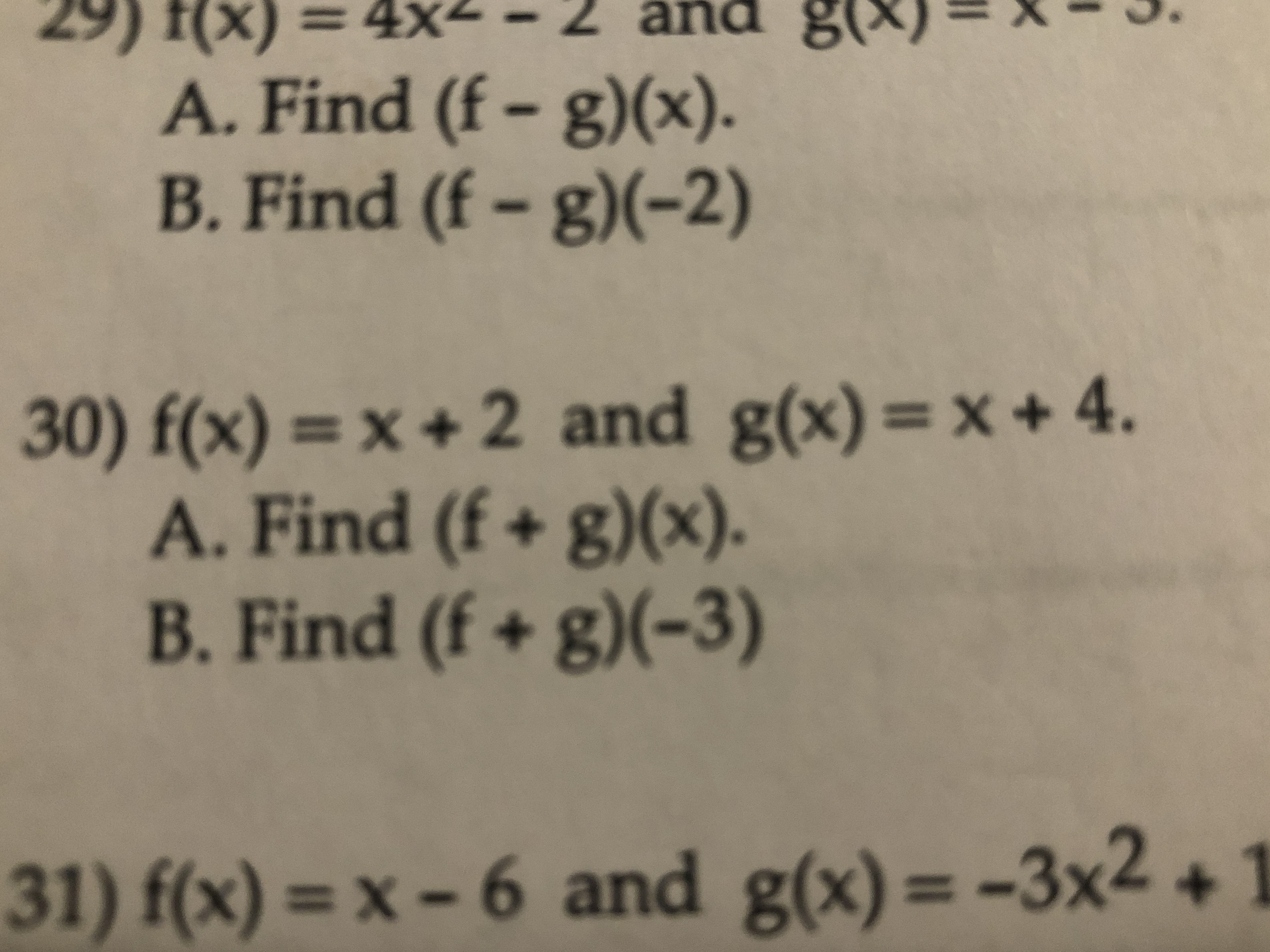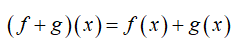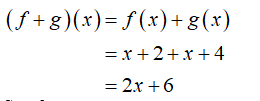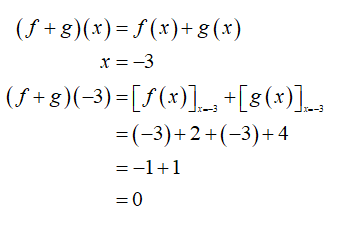# 29) f(x) = 4x2 – 2 añd g(×)=& - 3.A. Find (f - g)(x).B. Find (f - g)(-2)pue30) f(x) = x + 2 and g(x)=x+ 4.A. Find (f + g)(x).B. Find (f + g)(-3)31) f(x) = x – 6 and g(x) = -3x2 +

Question
2 views

30. Evaluatehelp_outlineImage Transcriptionclose29) f(x) = 4x2 – 2 añd g(×)=& - 3. A. Find (f - g)(x). B. Find (f - g)(-2) pue 30) f(x) = x + 2 and g(x)=x+ 4. A. Find (f + g)(x). B. Find (f + g)(-3) 31) f(x) = x – 6 and g(x) = -3x2 + fullscreen
check_circle

Step 1

Part A:

By the definition for operations on function:Step 2

Put the value of f(x) = x + 2 and g(x) = x + 4 in the above equation:Step 3

Hence, the value of the expression (f + g) (x) is 2x + 6.

Part B

Find the value of the expression (f + g) (x) at x = -3....

### Want to see the full answer?

See Solution

#### Want to see this answer and more?

Solutions are written by subject experts who are available 24/7. Questions are typically answered within 1 hour.*

See Solution
*Response times may vary by subject and question.
Tagged in